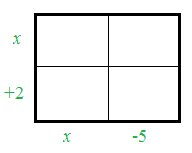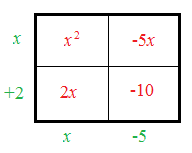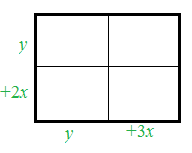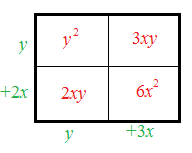### Home > AC > Chapter 6 > Lesson 6.2.3 > Problem6-66

6-66.

Use generic rectangles to multiply each of the following expressions.

1.  $( x + 2 ) ( x - 5 )$

• Draw a generic rectangle. A generic rectangle where the outside upper left edge is x and the lower left edge is + 2. The bottom left edge is x and the bottom right edge is negative 5.

• Multiply the value of the column and the value of the row to find the value of each box in the rectangle. A generic rectangle where the outside upper left edge is x and the lower left edge is + 2. The bottom left edge is x and the bottom right edge is negative 5. The interior is labeled as follows: Upper left box is x squared. Upper right box is negative 5 x. Lower left box is 2 x. Lower right box is negative 10.

• $(x+2)(x−5)=x^2−3x−10$1.  $( y + 2 x ) ( y + 3 x )$

• See the hints in part (a). A generic rectangle where the outside upper left edge is y and the lower left edge is + 2 x. The bottom left edge is y and the bottom right edge is positive 3 x.

• $(y+2x)(y+3x)=y^2+5xy+6x^2$  A generic rectangle where the outside upper left edge is y and the lower left edge is + 2 x. The bottom left edge is y and the bottom right edge is positive 3 x. The interior is labeled as follows: Upper left box is y squared. Upper right box is 3 x y. Lower left box is 2 x y. Lower right box is 6 x squared.1.  $( 3 y - 8 ) ( - x + y )$

• See the hints in parts (a) and (b).

 Generic rectangle left edge top$3y\$ interior top left blank interior top right blank left edge bottom$-8\$ interior bottom left blank interior bottom right blank left edge bottom$-x$ right edge bottom$+y$
1.  $(x-3y)(x+3y)$

• See the hints in part (a).

 Generic rectangle interior top left blank interior top right blank interior bottom left blank interior bottom right blank